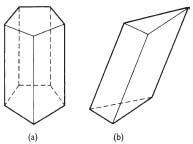Definition: prism from The Penguin Dictionary of Science

A ➤polyhedron formed by joining by straight lines corresponding points in two ➤congruent polygonal regions identically situated in parallel ➤planes. The volume of a prism is Ah, where A is the area of each polygon and h is the perpendicular distance between them. Prisms are named according to the shape of their polygonal faces; thus the familiar optical prism is a triangular prism.

Summary Article: prism
From The Penguin Dictionary of Mathematics

A solid figure formed from two congruent polygons with their corresponding sides parallel (the bases) and the parallelograms (lateral faces) formed by joining the corresponding vertices of the polygons. The lines joining the vertices of the polygons are lateral edges. Prisms are named according to the base - for example, a triangular prism has two triangular bases (and three lateral faces); a quadrangular prism has bases that are quadrilaterals.

Pentagonal, hexagonal, etc. prisms have bases that are pentagons, hexagons, etc.

A right prism is one in which the lateral edges are at right angles to the bases (i.e. the lateral faces are rectangles) - otherwise the prism is an oblique prism (i.e. one base is displaced with respect to the other, but remains parallel to it). If the bases are regular polygons and the prism is also a right prism, then it is a regular prism.prism (a) Right pentagonal and (b) oblique triangular prisms.Copyright © Penguin Books Ltd, 1989, 1998, 2003, 2008

### Related Articles

##### Full text Article prism
Philip's Encyclopedia

In mathematics, a solid geometrical figure whose ends are congruent (most commonly triangles) and perpendicular to the length, with the other...

##### Full text Article Prism
Illustrated Dictionary of Science, Andromeda

In geometry, a solid figure having two faces (the bases) which are parallel equal polygons and several others (the lateral faces) which are...

##### Full text Article regular prism
The Penguin Dictionary of Mathematics

A right prism that has regular polygons as bases. ...

See more from Credo